### Equivalence of Energy and Atomic Gravitation

###### Journal Name : SunText Review of Material Science

Article Type : Research Article

Authors : Orlov S

Keywords : Theory of vortex gravitation

### Abstract

Investigated directly proportional dependence of the energy end mass of a physical object on the strength of the vortex gravitation, which created the object.

### Earthworm Dynamics

Introduction

In 1900, Henri Poincare noticed that the radiation energy has a mass m, equal to the energy divided by the square of the speed of light: m = E/c2 . Later this relationship used by Einstein in his work on the principle of relativity. Since then, the claim that the mass can generate energy and vice versa has been called "The principle of the equivalence of mass and energy," and became a fundamental, which prohibit criticism. In the theory of vortex gravitation, cosmology and cosmogony , the evidence that the ether vortex rotation in space alter the pressure in it, create gravity and celestial bodies. In the paper the rationale that there are torsion bars not only on a cosmic scale, but also essential mikro torsion, the epicenter of which the pressure is several orders of magnitude smaller than in free ether. The sharp decrease in pressure causes a mikro torsion elementary particles. Relationship between the rotation and change of air pressure in the ether based on the principle of Bernoulli: changing the flow rate of a continuous medium is inversely proportional to the pressure in this thread. Therefore, the increase of the orbital velocity of ether causes a decrease in the torsion pressure towards the center of torsion.

### Energy and Vortex Gravitation

Pressure change in the gaseous environment, in addition to creating the forces pushing (gravity) and generates energy in a given volume of ether. This pattern is represented by the equation Mendeleev-Clapeyron. E = 3/2 PVt (1), where E - Energy, P - Pressure, Vt - volume of ether’s torsion. We will suppose that atoms are created ether’s mikro torsion. Orbital speed of rotation of ether in mikro torsion decreases to direction from a center to periphery by law of reverse square. On the basis of principle of Bernoulli of pressure in ether of micro torsion changes inversely proportional to speed of rotation of ether on the examined orbit. Consider the model of the atom in (Figure 1). Around the nucleus of the atom radius r1 rotates broadcast. Density of the nucleus of an atom is so large that air cannot penetrate it. Consequently, the surface of the core is lower barrier to rotation of the ether in torsion. Velocity v1 - the maximum, the orbital velocity of the ether. The pressure in the volume of gaseous medium depends on density of the medium and its speed – v1. According to the theory of vortex gravitation . Pressure is the orbital speed of ether the following relationship.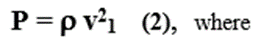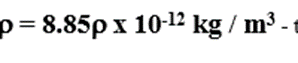The density of ether, an indication, according to .V1 - linear orbital velocity of the ether at the surface of the nucleus. Substituting (2) into (1)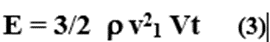To determine the rate of ether flow v in atomic torsion bars assume that the force of gravity vortex has little effect on the periphery of the torsion bar, order F? ~ 10-4 m. Based on the theory of vortex gravitation  with a force of gravity and orbit radius of the order of atomic torsion R ~ 10-7 , the linear velocity of the ether should be v ~ 109. For the dependence of v ~ r-1/2, it is determined that the surface of the nucleus, at r ~ 10-15 linear velocity is v1 ~ 1013

1.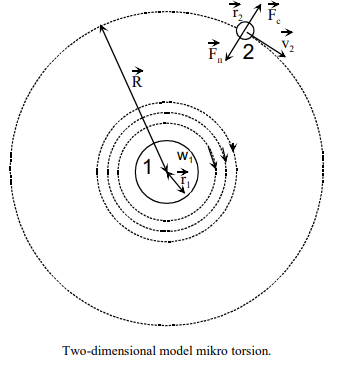2. F? - the force of gravity of the body 2 of the body 1

R - Radius of atomic torsion

r1 - radius of the nucleus of the atom 1

Substitute in equation (3) values of the following orders: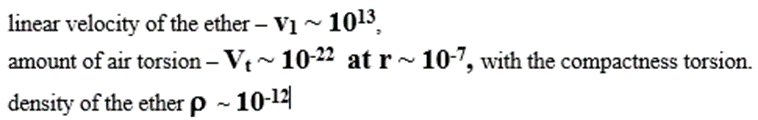We obtain the energy of the order of: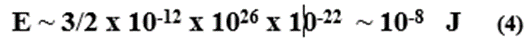To determine the energy in a certain volume of uranium of mass m, it is necessary to multiply the amount of energy of one atom determined by formula (4) by the number of atoms in this volume. The number of atoms is determined as the ratio of the mass of the substance to the mass of one atom ~ 10-25.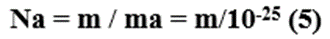Let us determine the energy in a body of mass m: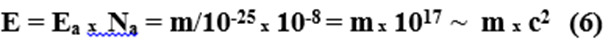CONCLUSION:

Based on the formula of Poincare define the order of the energy of the uranium atom in its mass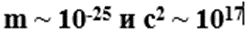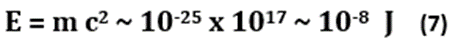Consequently, the value of one atomic energy torsion theory calculations of the vortex gravitation (Formula 3), corresponds to the energy of the atom defined by the classical, experimental formula. In the theory of vortex gravitation  that the gravitational torsion create body. That is, the mass of any celestial body depends on the speed of rotation of the corresponding torsion. This statement is valid for the mass of a particle of air speed in the corresponding micro torsion. Thus, on the basis of the theory of gravity vortex can conclude - the energy and mass of one body created by the same process - gravitational torsion. We can therefore say that the mass of a body (atom) does not create the energy of the body (an atom). To the extent it can be argued that the energy of the body does not produce mass. These physical properties of a body are each only an indirect relationship, as they are generated by the same rotation of the vortex ether. At fracture (splitting) of the atom (nucleus) breaks down and the nuclear torsion. The pressure in the torsion increases sharply to values of free ether, which should be accompanied by a release of energy, according to the formula (3).